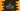# C program to print the addition table for a number### C program to print addition table for a number :

In this C programming tutorial, we will learn how to print addition table for a specific number . For that, the user will enter one number and we will read and save that number . After that using one loop, the program will print one addition table. For example, the addition table for 5 will loop like as below :

``````5 + 1 = 6
5 + 2 = 7
5 + 3 = 8
5 + 4 = 9
5 + 5 = 10
5 + 6 = 11
5 + 7 = 12
5 + 8 = 13
5 + 9 = 14
5 + 10 = 15``````

We will learn to solve this problem by using a for loop and by using a while loop. Let’s take a look at the programs :

``````#include

int main(){
//1
int number;
int i;

//2
printf("Enter the number : ");
scanf("%d",&number);

//3
for(i=1 ;i<=10 ;i++){
//4
printf("%d + %d = %d\n",number ,i ,number + i);
}

}``````

### Explanation :

The commented numbers in the above program denote the step number below :

1. Create one variable number to hold the user input number and one variable i to use in the loop below.
2. Ask the user to enter the number . Using scanf, store the user input value to number variable.
3. Run one for loop. This loop will run from i = 1 to i = 10 and increment the value of i by 1.. For each iteration of this loop, print one line of the addition table.
4. This printf will print the addition table line. First %d will print the value of user given number, second %d will print the current value of i and the third %d will print the value of summation of both values i.e. number + i.

### Sample Output :

``````Enter the number : 6
6 + 1 = 7
6 + 2 = 8
6 + 3 = 9
6 + 4 = 10
6 + 5 = 11
6 + 6 = 12
6 + 7 = 13
6 + 8 = 14
6 + 9 = 15
6 + 10 = 16``````
``````Enter the number : 7
7 + 1 = 8
7 + 2 = 9
7 + 3 = 10
7 + 4 = 11
7 + 5 = 12
7 + 6 = 13
7 + 7 = 14
7 + 8 = 15
7 + 9 = 16
7 + 10 = 17``````

### Using a while loop :

We can achieve the same result by using a while loop also. Take a look at the below program :

``````#include

int main(){
int number;
int i;

printf("Enter the number : ");
scanf("%d",&number);

i = 1;
while(i<=10){
printf("%d + %d = %d\n",number ,i ,number + i);
i++;
}

}``````

Here , instead of a for loop, we are running one while loop to print the addition table. The printf statement is same as above. Inside the while loop, we are incrementing the value of i each time. It starts from 1 and ends at 10. Same as above. The output of this program will look something like below :

``````Enter the number : 9
9 + 1 = 10
9 + 2 = 11
9 + 3 = 12
9 + 4 = 13
9 + 5 = 14
9 + 6 = 15
9 + 7 = 16
9 + 8 = 17
9 + 9 = 18
9 + 10 = 19``````